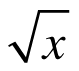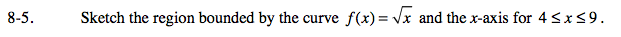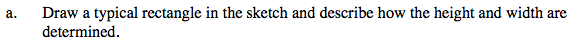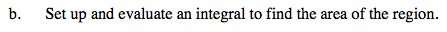### Home > CALC > Chapter 8 > Lesson 8.1.1 > Problem8-5

8-5.
1. Sketch the region bounded by the curve f(x) =and the x-axis for 4 ≤ x ≤ 9. Homework Help ✎

1. Draw a typical rectangle in the sketch and describe how the height and width are determined.

2. Set up and evaluate an integral to find the area of the region.Δx is the width, and f(xxi) is the heightas Δx→0, f(axi)→__________